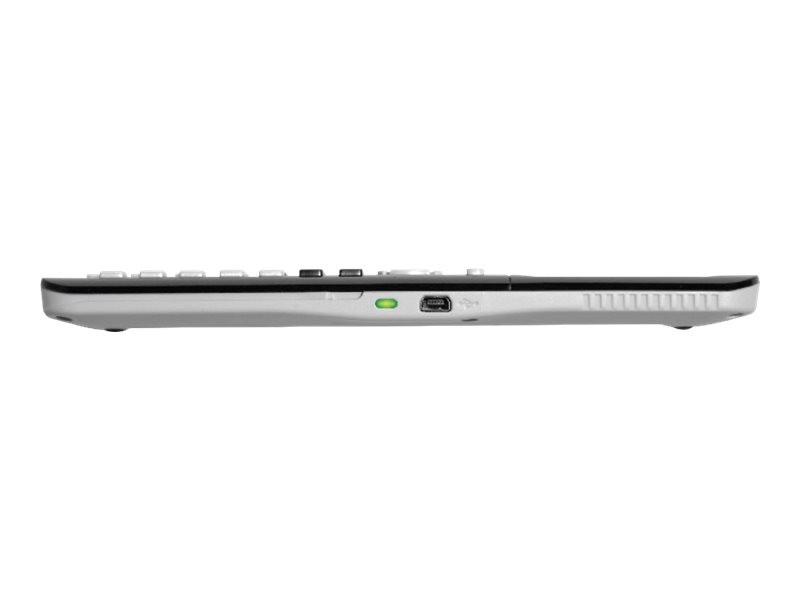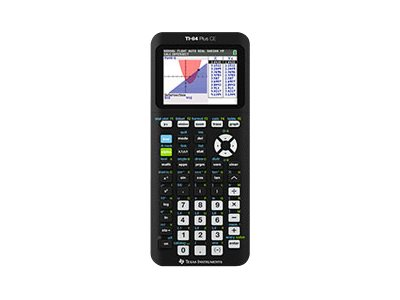BrowseBrowse by Category
Browse by Category
Help
•••# Texas Instruments - Texas Instruments TI-84 Plus CE

Graphing calculator - USB - 10 digits + 2 exponents - battery - black

Texas Instruments
84PLCE/TBL/1L1
0191081807227
N/A
New

## Price: \$177.46

Total Available: 422

## Specifications

Main Specifications
Product Description Texas Instruments TI-84 Plus CE - graphing calculator
Product Type Graphing calculator - LCD - 10 digits + 2 exponents
Interface USB
Basic Functions Addition, subtraction, multiplication, division
Scientific Functions Complex numbers, statistics, statistical analysis, linear regression, hypothesis testing, confidence intervals, variables, linear equation, matrix, logarithmic regression, exponential regression, power regression, integral, polynomial, derivatives, real numbers, internal precision (14 digits), inverse matrix, matrix to list conversion, quadratic polynomial regression, cubic polynomial regression, quartic polynomial regression, median-median regression, logistic regression, sinusoidal regression, analysis of variance, polynomial root finder, probability
Financial Functions Amortization, cash flow, Time Value of Money (TVM)
Graphing Functions Polar, sequence, zoom, parametric plot, histogram, scatter plot, cobweb plot, stairstep plot, box and whisker plot, normal probability, transformation, conic, inequality plot, rectangular, recursion, statistical plot, phase plot
Diagonal Size 2.8"
Color Support Color
Power Source Battery
Color Black
Features Clock, calendar, programmable, upgradable firmware
General
Product Type Graphing calculator
Interface USB
Power Source Battery
ROM 3 MB
RAM 154 KB
Features Clock, calendar, programmable, upgradable firmware
Miscellaneous
Color Black
Included Accessories Hard case, unit-to-unit USB cable
Warranty 1 year
Software
Software Included Cabri Jr., CellSheet, Vernier EasyData, Polynomial Root Finder and Simultaneous Equation Solver, Conic Graphing, Inequality Graphing, Probability Simulation, Transformation Graphing App
Display
Type LCD - 10 digits + 2 exponents
Diagonal Size 2.8"
Color Support Color
Display Resolution 320 x 240 pixels
Functions